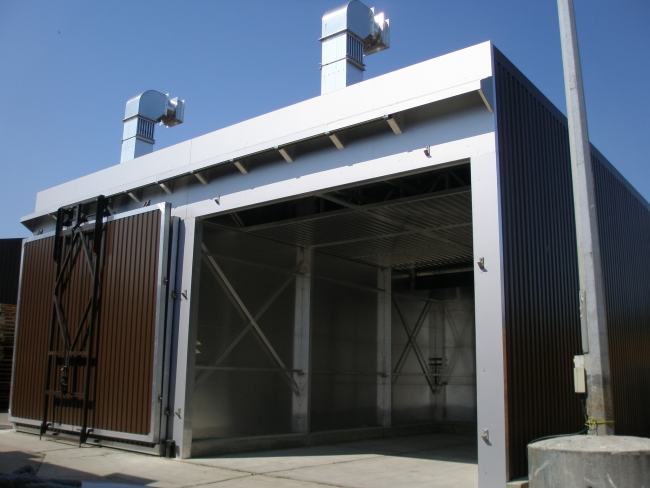Manufacturers of Timber, Drying Kilns for over 35 years 01621 785 935info@kilnservices.co.uk

# Heat Recovery

Timber drying requires a considerable amount of energy to evaporate the large volume of water held in the wood.

A kiln drying 160 cubic metres of Sitka Spruce, with a dry weight density of 410 kg per cubic metre, from an initial moisture content of 60% down to 18% will need to remove / evaporate 173 litres / kg of water from each cubic metre of wood. i.e. 27,680 Litres or 27.68 tonnes of water per 160 cubic metres dried.

The total heat energy used would be around 255 kw/hrs per cubic metre or 40,800 kw/hrs over a 4 day drying cycle. If using oil this would be around 3,800 litres.

To calculate the energy saving from heat recovery we need to know how many cubic metres of air need to be vented to the atmosphere to remove the 27.68 tonnes of water held in the wood.

If we assume the fresh inlet air has a temperature of 15° Centigrade and 60% Relative Humidity, each cubic metre of fresh air entering the kiln will hold around 7.73 grams of water.

Assuming average drying conditions within the kiln of 65° Centigrade and 38% Relative Humidity, each cubic metre of vented air will hold around 61 grams of water.

If we deduct the moisture in the inlet air from the exhaust air we get an extraction rate of 53.27 grams of water per cubic metre.

Therefore, to remove 27,680 litres of water we would need to vent 519,617 cubic metres of air over a 4 day drying cycle.

Example calculation:

Volume of Air Vented / Second = 2 Cubic Metres multiplied by
Specific Heat of Air = 1.2 multiplied by
Temperature increase of Inlet Air = 32°Centigrade
Gives a heat transfer of 76.80 kw/hrs per hour

Venting time to remove 27.68 tonnes = 72 Hours multiplied by
Heat transfer per hour 76.80 kw/hr
Total heat transfer per each 160 cubic metre batch load = 5,529 kw/hrs

The total electrical energy used by the heat recovery system in the kiln shown would be approximately 510 kw/hrs.Two 50 cubic metre capacity kilns each with a small heat recovery system. The heat recovery system can be supplied in three sizes according to your specific requirement.View of heat recovery system in operation.Inside of the heat recovery system showing the large surface area of the aluminium extruded fins that transfer the heat from the exhaust to the inlet ducts.

### Quick Contact

Website Design by SiteWizard